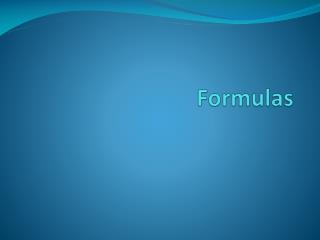Download PresentationFormulas

Formulas - PowerPoint PPT Presentation

Formulas. Heat Loss due to Infiltration & Ventilation. Infiltration 1.1 x [(ACH x vol.) /60] x (Ti -To) -or- .018 x ACH x Vol. x (Ti- To) Note: CFM = (ACH x volume) / 60 min per hour Ventilation 1.1 x [( R a x SF) + (No. of people x R p )] x (Ti –To).I am the owner, or an agent authorized to act on behalf of the owner, of the copyrighted work described.
Download PresentationFormulas

Download Policy: Content on the Website is provided to you AS IS for your information and personal use and may not be sold / licensed / shared on other websites without getting consent from its author.While downloading, if for some reason you are not able to download a presentation, the publisher may have deleted the file from their server.

- - - - - - - - - - - - - - - - - - - - - - - - - - E N D - - - - - - - - - - - - - - - - - - - - - - - - - -
Presentation Transcript
1. Formulas

2. Heat Loss due to Infiltration & Ventilation Infiltration 1.1 x [(ACH x vol.) /60] x (Ti -To) -or- .018 x ACH x Vol. x (Ti- To) Note: CFM = (ACH x volume) / 60 min per hour Ventilation 1.1 x [( Ra x SF) + (No. of people x Rp)] x (Ti –To)

3. Heat Loss Due to Infiltration Infiltration Please Note: For tight construction use 0.5 for ACH. For medium construction use .85 for ACH. For loose construction use 1.3 for ACH. For really bad construction use 2.0 for ACH For the summer months (cooling) use 70% of the winter values.

4. Heat Gains from Occupant Loads Sensible per occupant X number of occupants = Btu h Latent per occupant X number of occupants = Btu h

5. Heat Gains from Infiltration Loads Sensible Btu h = 1.1 x CFM x TD Latent Btu h = 4,500 x CFM x (Wroom – Woa)

6. Heat Gains from Outside Air for Ventilation Loads Sensible Btu h = 1.1 x CFM x TD Latent Btu h = 4,500 x CFM x (Wroom – Woa) -or- Btu h = .68 x CFM x (W2 – W1)

7. Degree Days for Pullman • HDD = 6655 • CDD = 1154 Degree Day = 65°F - ((high temp. – low temp])/2)

8. Formula Operating Hours = [Degree Days x 24] / [ Temperature Difference] Heating Degree Days for Pullman = HDD = 6655 Temperature Difference =ΔT (for project)

9. Formula Operating Hours = [Degree Days x 24] / [ Temperature Difference] Cooling Degree Days for Pullman = CDD = 1154 Temperature Difference =ΔT (for project)

10. Estimating Annual Energy Heating \$/Yr (BTU/Hr) * (hours of operation) * \$/energy unit BTU/energy Unit * Efficiency Note: Does not include fan energy. Add 10% for residential and 20% for commercial fan energy

11. Estimating Annual Energy Cooling \$/Yr (Btu/Hr) * (hours of operation) * \$/energy unit SEER * 1000 Note: Does not include fan energy. Add 10% for residential and 20% for commercial fan energy

12. Heating Cost for Operating Hours = [Degree Days x 24] / [ Temperature Difference] [6,655 x 24]/87 = 1836 Operating Hours = 1,836

13. Electrical Cost to Heat [(BTU/Hr) * (hours of operation) * \$/energy unit]/ BTU/energy Unit * Efficiency .08 electrical cost per kilowatt hours [(2,310,240) * (1,836) * .08]/ (3,400 * 1 .00) = \$99,802.37